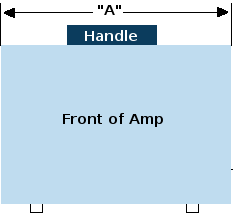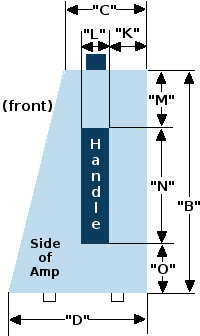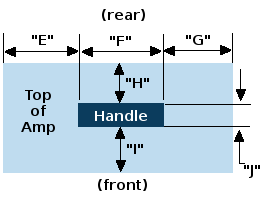Combo with Angled Baffle and 3 Handles

• inches
• mm
•• inches
• inches
• inches
• mm
• mm
• mm
•• inches
• inches
• inches
*NOTE The dimensions for E + F + G must add up to the measurement for A
• inches
• inches
• inches
*NOTE The dimensions for H + I + J must add up to the measurement for C
• inches
• inches
• inches
• inches
• inches
*NOTE The dimensions for M + N + O must add up to the measurement for B
• mm
• mm
• mm
*NOTE The dimensions for E + F + G must add up to the measurement for A
• mm
• mm
• mm
*NOTE The dimensions for H + I + J must add up to the measurement for C
• mm
• mm
• mm
• mm
• mm
*NOTE The dimensions for M + N + O must add up to the measurement for B
•Brands

//begin script sum text